This is series of blog posts of me trying to share what I’ve explored in the course ‘From Deep learning foundations to Stable Diffusion’ by FastAI.

Everything I share in this post is based on the initial lessons of the FastAI course, which is publicly available here. The lessons also have some accompanying jupyter notebooks on stable diffusion, which can be found in this Github repo.

Let’s jump right into the post with the question: ‘What is stable diffusion?’.

## What is stable diffusion?#

In simple words, stable diffusion is a deep learning model that can generate an image given a prompt as shown in the figure below: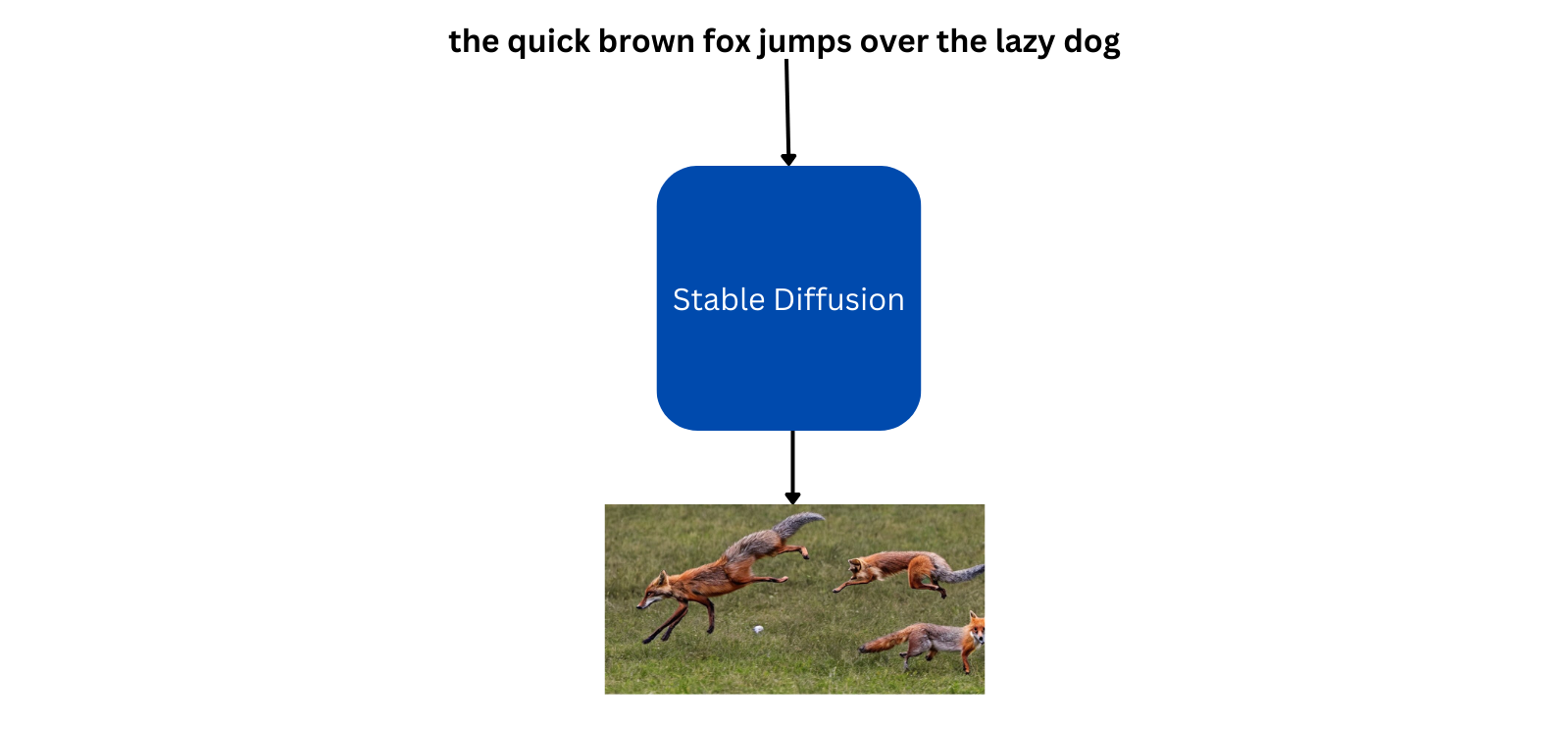It can also take an image as a starting point along with a prompt, to generate an image similar to the input image. For example, if the model is given a random drawing of some animal looking at the moon along with the prompt ‘wolf howling at the moon’, the model will generate a good looking image with similar setting as the input image: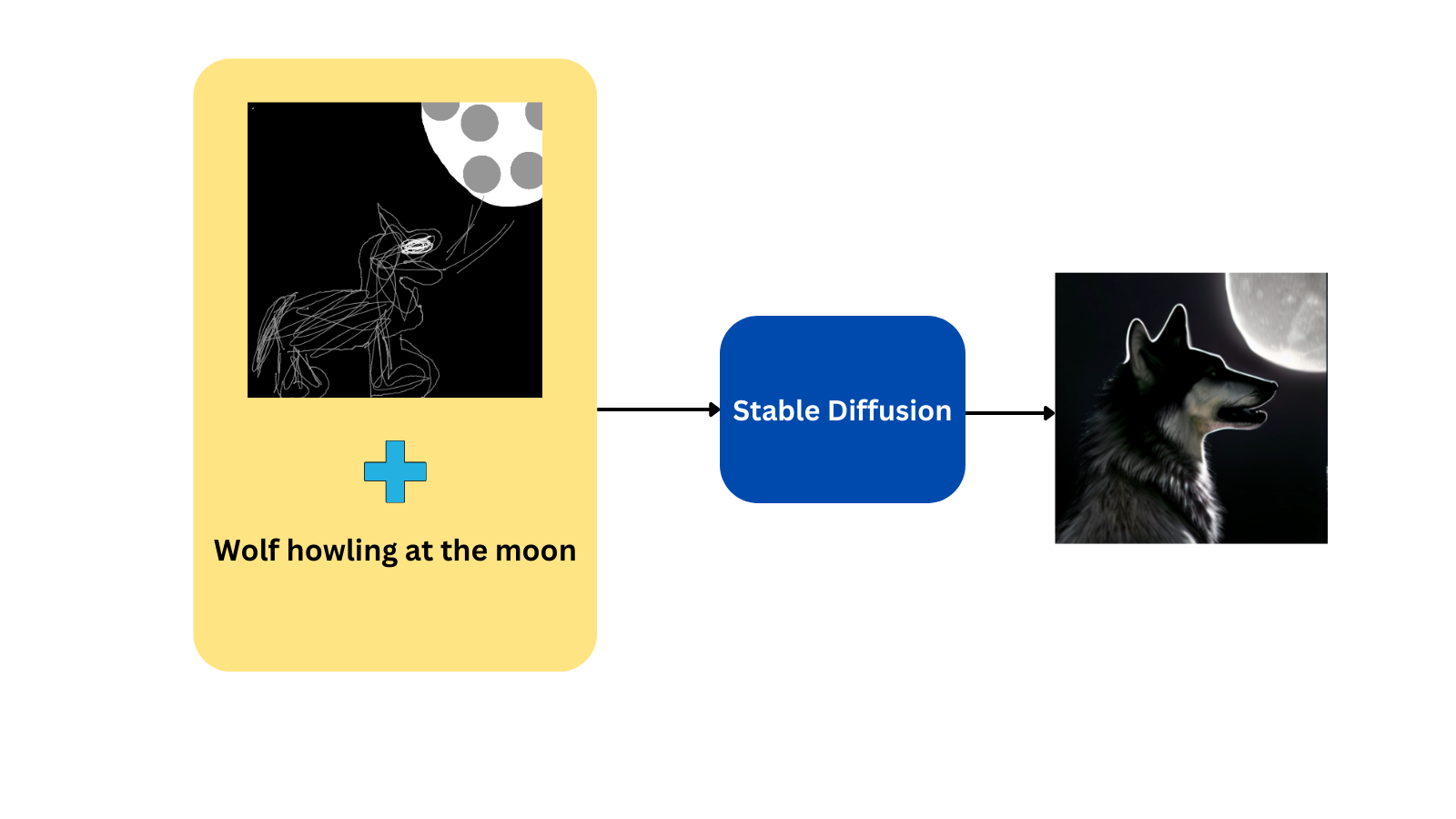## But how does this whole thing work?#

First, let’s see how the model generated an awesome wolf image from a random sketch and a simple prompt. This generation process involves 3 different models:

1. A model for converting the text prompt to embeddings. Openai’s CLIP(Contrastive Language-Image Pretraining) model is used for this purpose.
2. A model for compressing the input image to a smaller dimension(this reduces the compute requirement for image generation). A Variational Autoencoder(VAE) model is used for this task.
3. The last model generates the required image according to the prompt and input image. A U-Net model is used for this process.

We will summarize each of the process above using simple diagrams:

### CLIP Embeddings#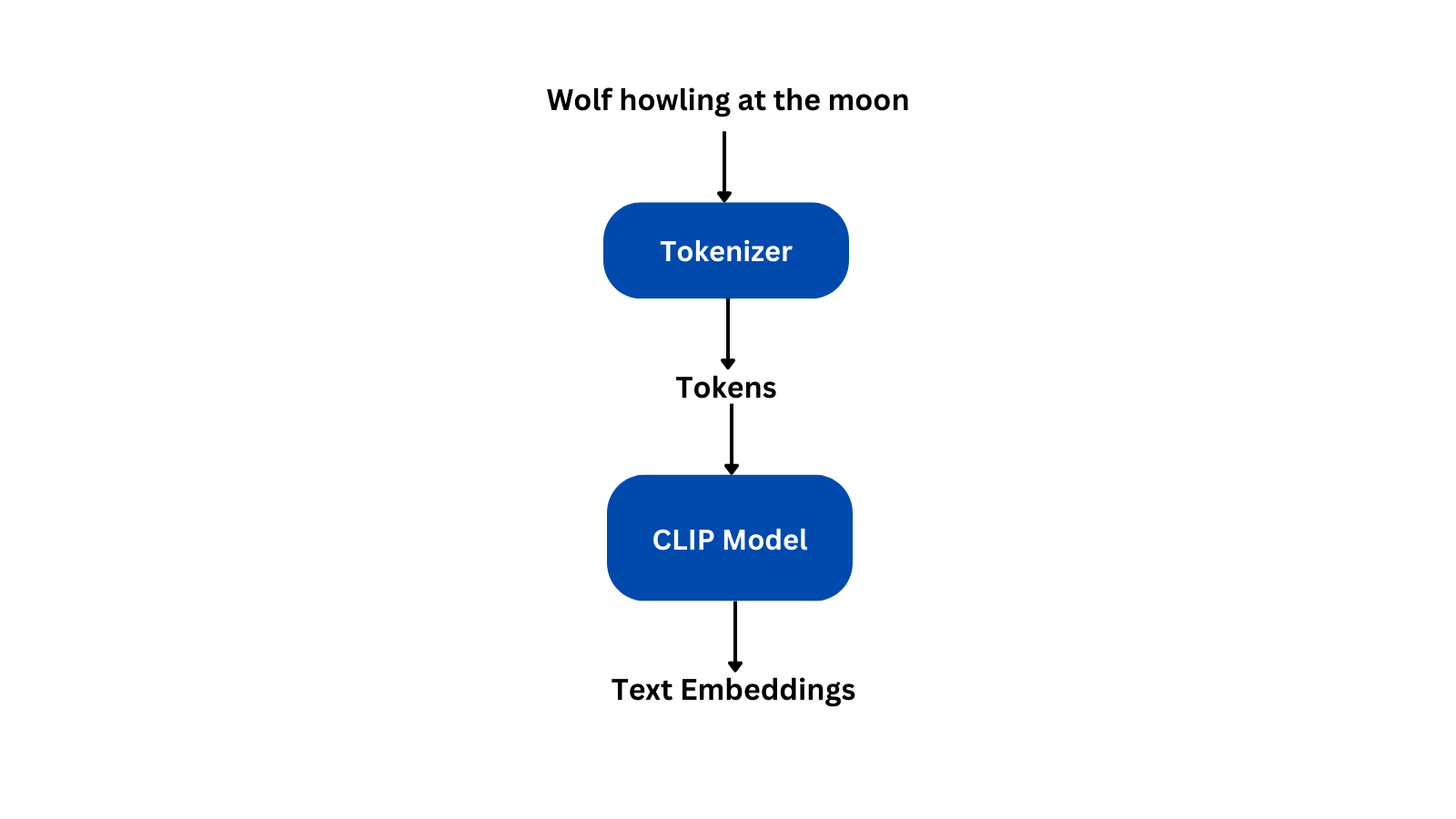As seen in the above figure, CLIP tokenizer converts the prompt into tokens and feeds it into the CLIP model to get the text embeddings.

### Encoder part of VAE model#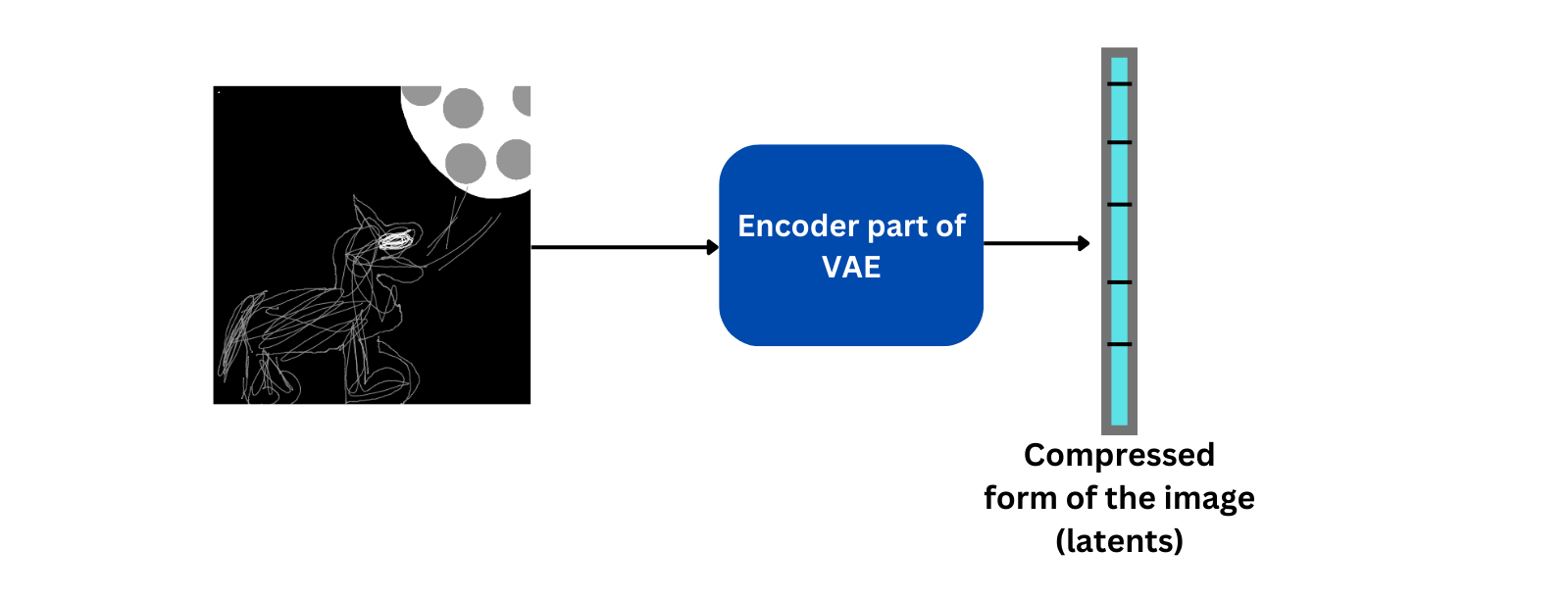The VAE model has an encoder as well as a decoder part. Given an image, the encoder part of the VAE compress the image to a low dimension tensor without much loss in information. This compressed form is called latents. The decoder is used to recover the original image from the latents. In the above figure, only the encoder part is shown. We will talk more about decoder in the coming steps.

### U-Net model#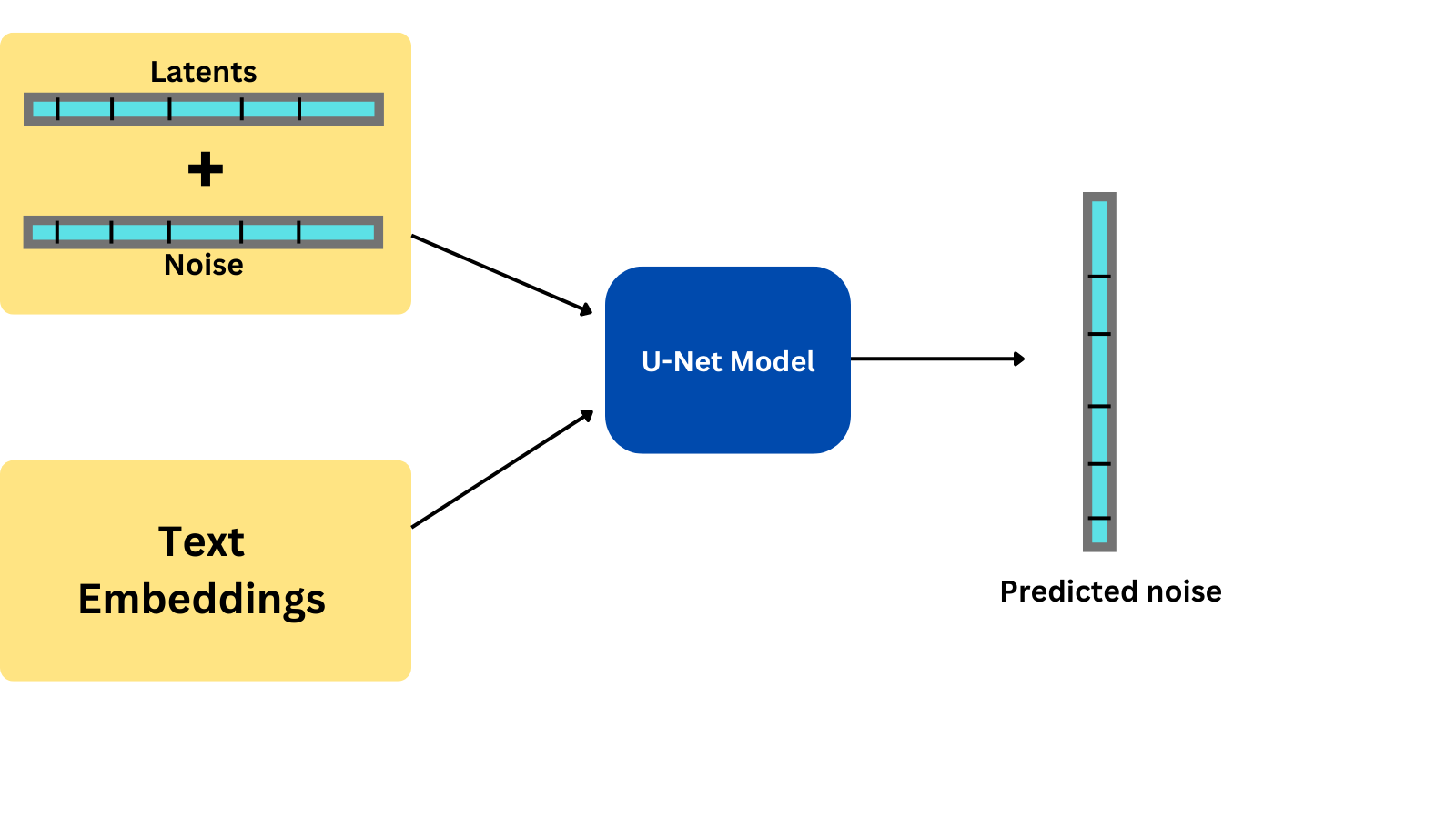The U-Net model takes in the latents created by VAE as well as the text embedding created by the CLIP model as inputs. But before we pass in the latents, we add some noise to it. Thus, the U-Net model does not copy the input image as it is.

With the noisy latents combined with text embeddings as inputs, the U-Net model tries to predict the noise present in the latents. We subtract this predicted noise from the latents to get the original latents. We repeat this process a specified number of times, say for example, 50 times. During each iteration, the amount of noise added to the latents is reduced. That is, for each iteration, the amount of noise added to the latents will be less than the previous iteration.

### Decoder part of VAE model#

Once the specified number of iterations is over, we get some latents that is ready to be decoded to an image. For this, we use the decoder part of the VAE model as shown below: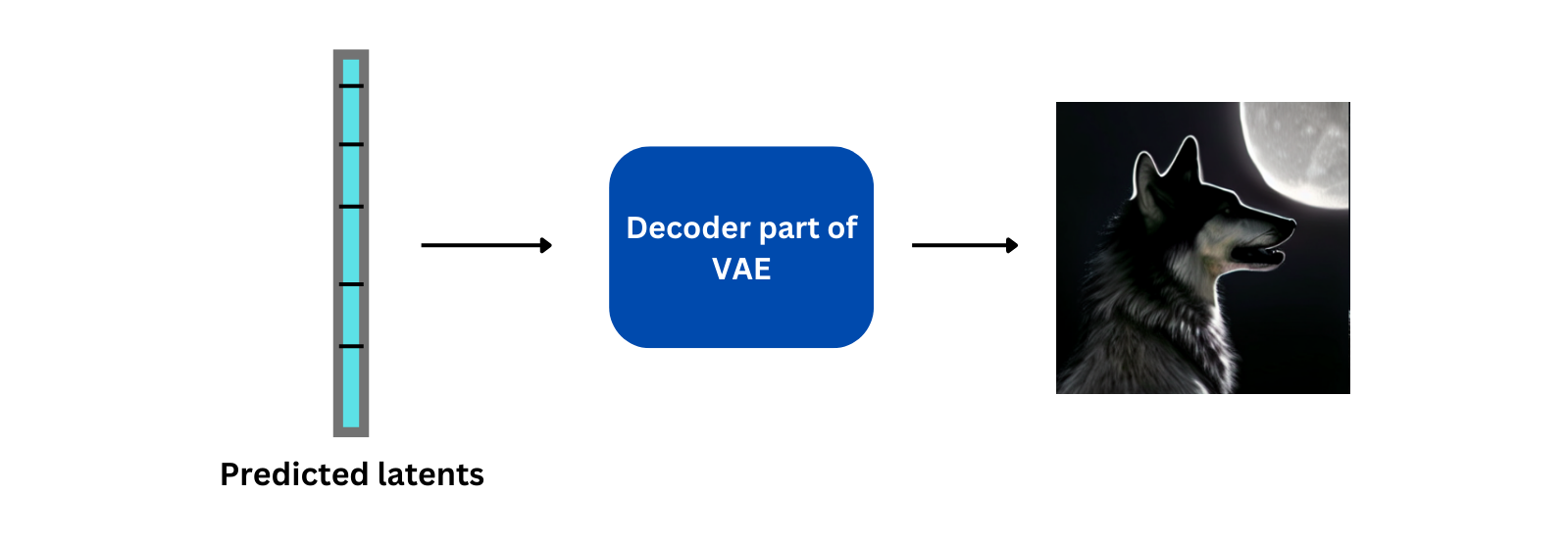This is a simplified version of how stable diffusion model generates an image given a prompt and an initial image. Since these models work on latent space rather than the original image, these types of models are also called latent diffusion models.

As said earlier in this post, the stable diffusion model can generate images even if we only provide a prompt describing the image we want. The above pipeline remains the same for this task also, but the only difference is that we don’t require the encoder part of the VAE model as we dont have any initial images to encode. So we just create a random tensor with the same size as the latents to feed to our U-Net model.

## Looking into the source code of huggingface diffusers#

### Playing around with diffusers#

The diffusers library from huggingface already has implementations of the stable diffusion model and the library makes it easier to play around with these types of models.

Before you can play around with these models, you need to go here and accept the terms and conditions. Once you do that, you need to create a new token from here and copy it into the box that appears when you run the following code in your jupyter notebook:

``````!pip install huggingface-hub==0.10.1

``````

Once everything is completed successfully, install the dependencies(as of writing this post, the latest version of diffusers and transformers are `0.6.0` and `4.23.1` respectively):

``````!pip install -qq -U diffusers transformers
``````

Generating images with diffusers and stable diffusion is as simple as running these 4 lines of code(the code requires a GPU machine):

``````from diffusers import StableDiffusionPipeline

# set up the pipeline to generate images
pipe = StableDiffusionPipeline.from_pretrained('CompVis/stable-diffusion-v1-4').to('cuda')

prompt = "a photograph of an astronaut riding a horse"
# generate an image for the above prompt
pipe(prompt).images
``````

If you wish to play around with the diffusers pipeline for image generation, do check out their documentation here.

### Diving deeper into `StableDiffusionPipeline`#

Now let’s look into the source code and see what exactly is going on when we pass the prompt to our pipeline. The exact code that I’m discussing here can be found here.

First, let’s learn more about the arguments passed to the stable diffusion pipeline: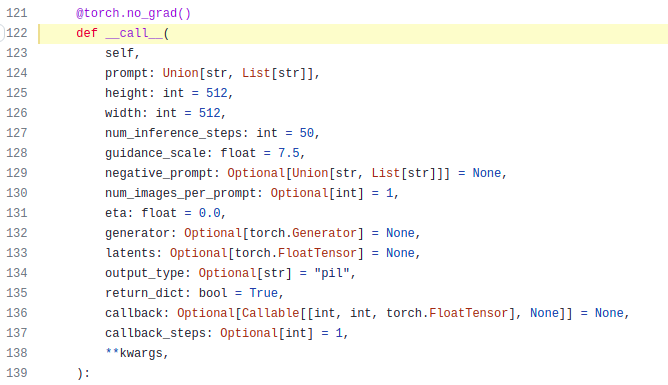Other than the prompt, there are a bunch of other arguments too. We can customize the image generation process by changing the values of these arguments.

Let’s check each one them and see how they will help us:

1. `prompt` - this is the prompt that we pass to our pipeline as in `pipe(prompt)`.
2. `height`, `width` - these are the dimensions of the generated image.
3. `num_inference_steps` - as we’ve discussed before, the U-Net model runs multiple times before generating the final latents. This is the denoising step. This argument defines the number of times to run the denoising step.
4. `guidance_scale` - guidance scale is the value that decides how close our image should be to the prompt. This is related to a technique called Classifier-Free Guidance(CFD), which improves the quality of images generated. Higher values will generate images close to the prompt but the quality may not be the best.
5. `negative_prompt` - this is another argument that takes in a prompt as input. But as the name suggests, this is a negative prompt. Thus, the model will try to generate images that doesn’t have any similarities with this prompt. As an example, if we provide ‘blue color’ as a negative prompt, the model will try to avoid including the color blue in the generated image.
6. `num_images_per_prompt` - the number of images to generate for a given prompt.
7. `callback` - this is mostly a callable function, that allows you to add some custom functionality into the pipeline.
8. `callback_steps` - number of times the callback function is to be called.

The documentation page of diffusers has all these arguments listed with details included.

Now let’s move on to the improtant parts of the pipeline. First let’s see how the text encoding part works: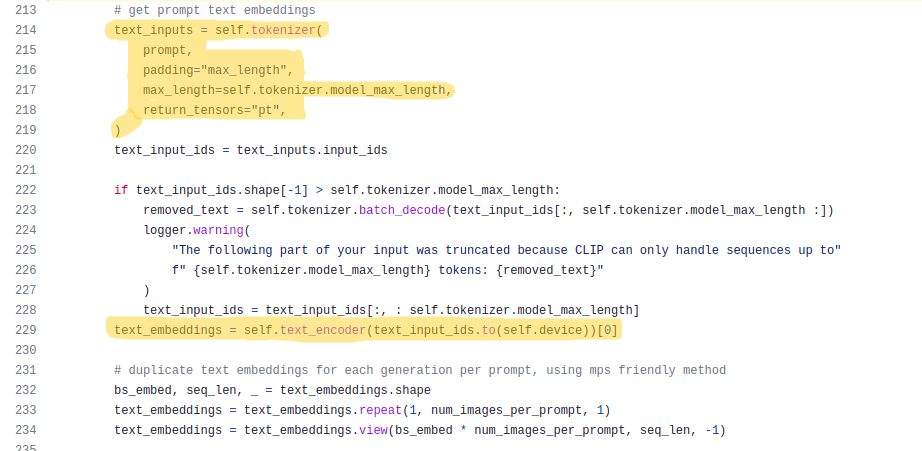In the above image, the highlighted part at the top contains the code for tokenizing. The prompt is tokenized, padded to the maximum length of the model and converted to pytorch tensors using a tokenizer. The second highlighted part is where we pass these tokens to the CLIP model(`text_encoder` in the code) to get the text embeddings.

Now let’s look into some code for doing classifier-free guidance: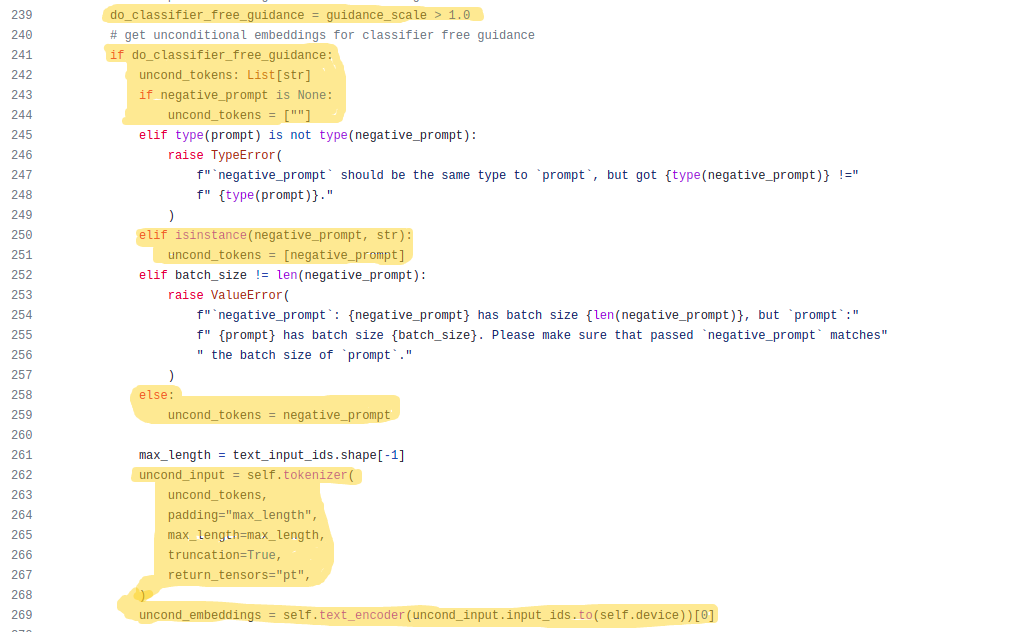Classifier free guidance is done only if the guidance scale is greater than 1, the highlighted part at the top(line `239`) checks this condition.

For doing classifier guidance, we need a new prompt called unconditional prompt which is an empty string(line `244`) if no value is passed to `negative_prompt`, otherwise, the same negative prompt is used as unconditional prompt(line `251` & line `259`).

After deciding on the unconditional prompt, the prompts are tokenized(line `262`) in the same manner as the normal prompts and encoded using the CLIP model(line `269`).

Once we are ready with the unconditional embedding and the text embedding, we concatenate them and move on to the next step: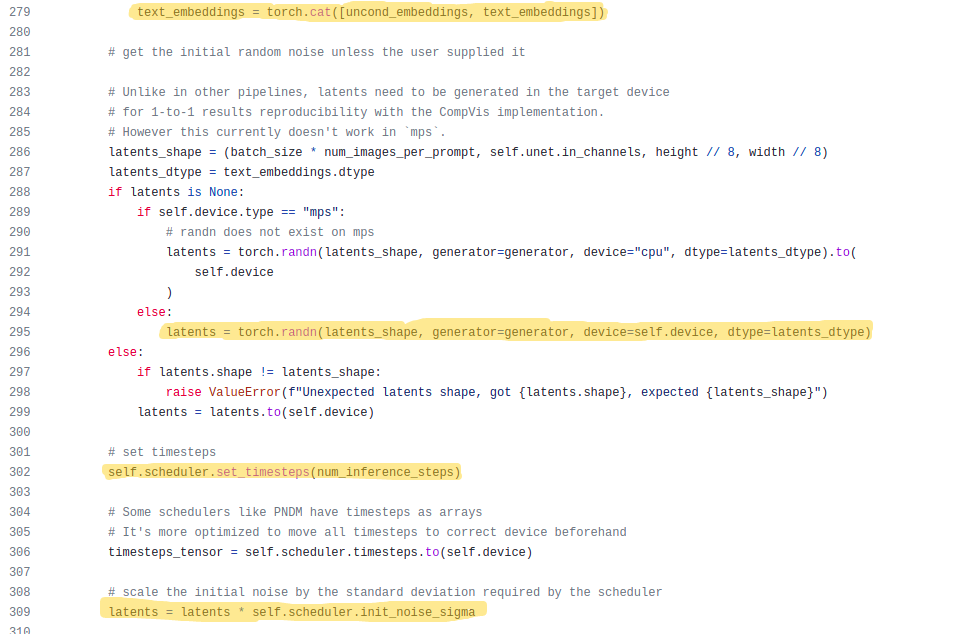The concatenation part is done in the first highlighted part. Once we have the embedding part of our input ready, we need to prepare the second input, that is the latents. For that we just create a random tensor(line `295`) with the same shape as the latents created by our VAE model for an image.

We have a scheduler which decides the amount of noise to be added to the latent at each iteration, so we give it the information regarding the maximum number of iterations possible(shown in line `302`).

As said earlier we add noise to the latents before passing it to the U-Net model(shown in line `309`).

Everything is set, now it’s time to enter the generation step: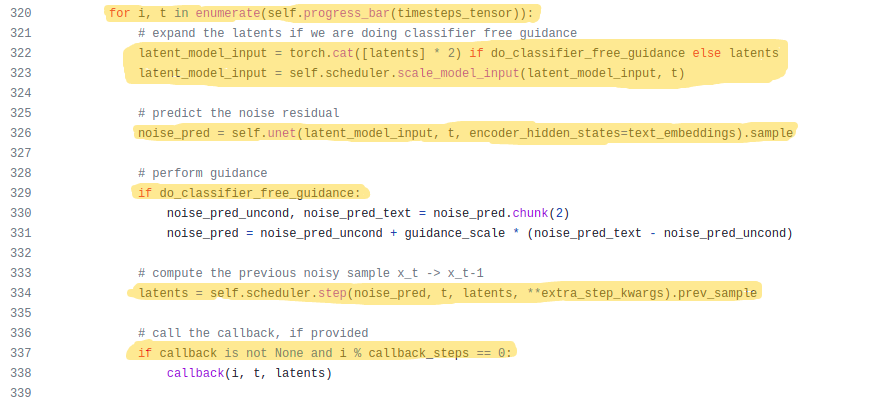In the generation process, we iterate till the maximum number is reached(line `320`). If we are doing classifier free guidance, we need to duplicate our latents(line `322`) because we have an unconditional embedding and a text embedding. So in order to generate one image for each of these embeddings, we need separate latents for them.

In the next two lines of code, we scale the latents as required by the U-Net model and the model predicts the noise present in the input latents. Thus if we are doing classifier free guidance, the model will output two latents, otherwise, only one.

For classifier free guidance, we get the predicted noise using the follwoing formula(line `331`):

``````predicted_noise = noise_predicted_for_unconditional_embedding + guidance_scale*(noise_predicted_for_text_embedding - noise_predicted_for_unconditional_embedding)
``````

The above equation is where the guidance scale comes into play. It has a huge effect on the predicted noise subtracted from our latents.

Finally, we pass the predicted noise and the current latents to our scheduler to prepare the latents for the next iteration(line `334`).

A new feature that was added recently is the callback function. If we pass any arguments to the `callback` argument, it gets executed inside the pipeline at line `338`. For example, let’s create a simple function to pass into `callback` argument:

``````def sample_function(i, t, latents):
# callback function should expect the above 3 arguments
print(i, t)
``````

Now if we pass this function to the pipeline as `pipe(prompt, callback=sample_function, callback_steps=3)`, then the function will be executed 3 times and each time it will print the values of `i` and `t`.

At last, we’ve reached the end of the pipeline source code. This is where the latents are coverted to image format using the decoder part of VAE model and finally returned as a PIL image, as shown in the highlighted part below: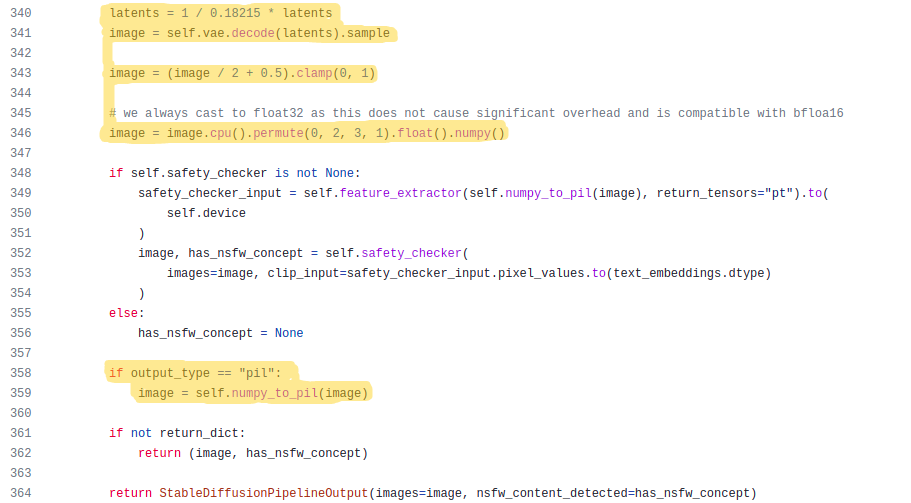The code that is in between the highlighted part(line `348` to `356`) checks the generated image for NSFW(Not Safe For Work) content. If this type of content is detected, the pipeline returns a black image(as of the current version of the library - `v0.6.0`).

## Concluding thoughts#

This is just an overview of what’s happening inside diffusion models and the stable diffusion pipeline in diffusers.

The FastAI course lectures and accompanying notebooks listed at the top of this blog post has some great explanations on the topics discussed so far. The notebooks also contain code to do some cool tricks using stable diffusion.

If you have any queries or want to discuss something machine learning, you could reach out to me via twitter @bkrish_.